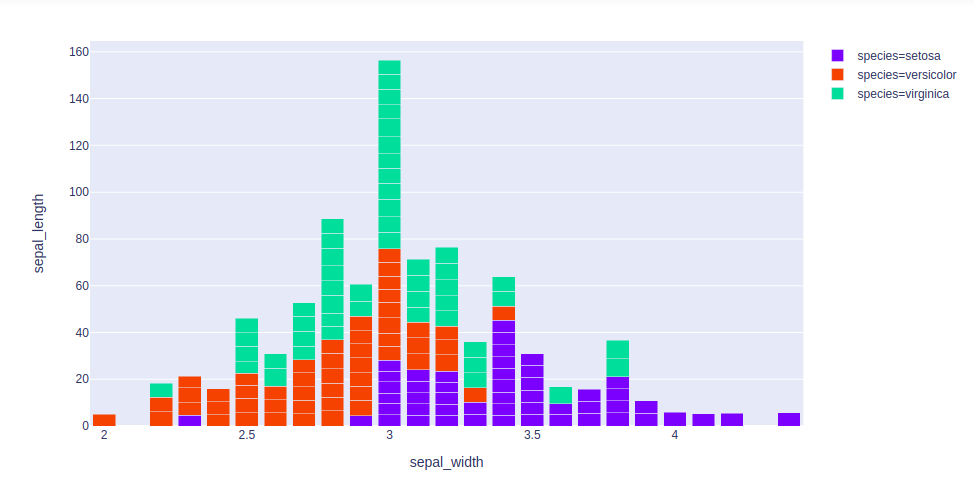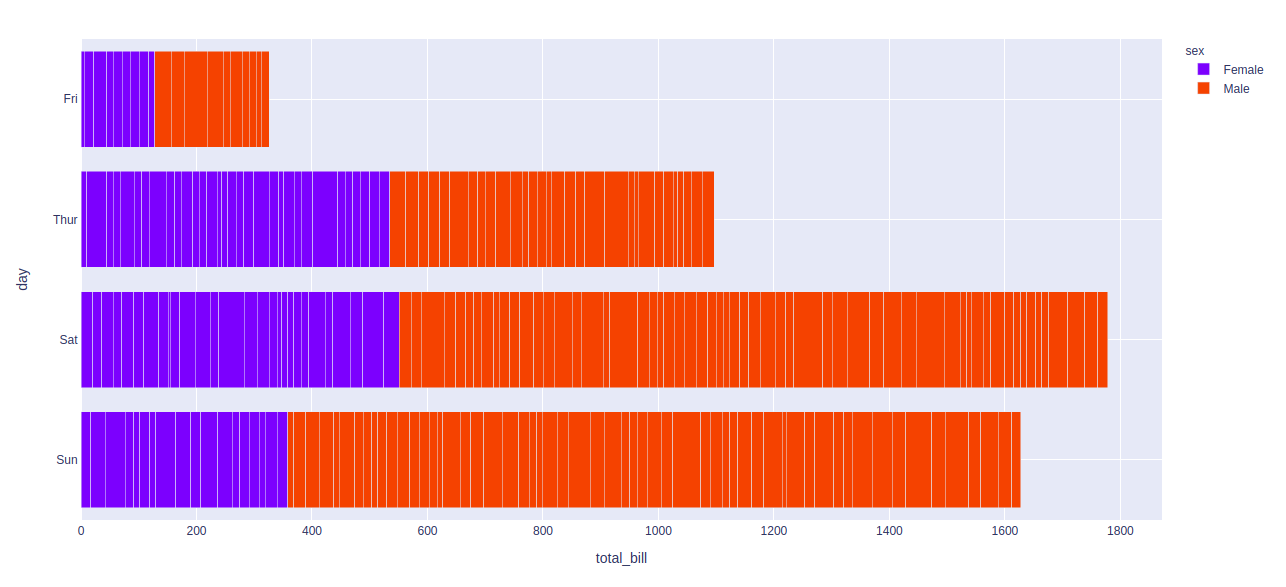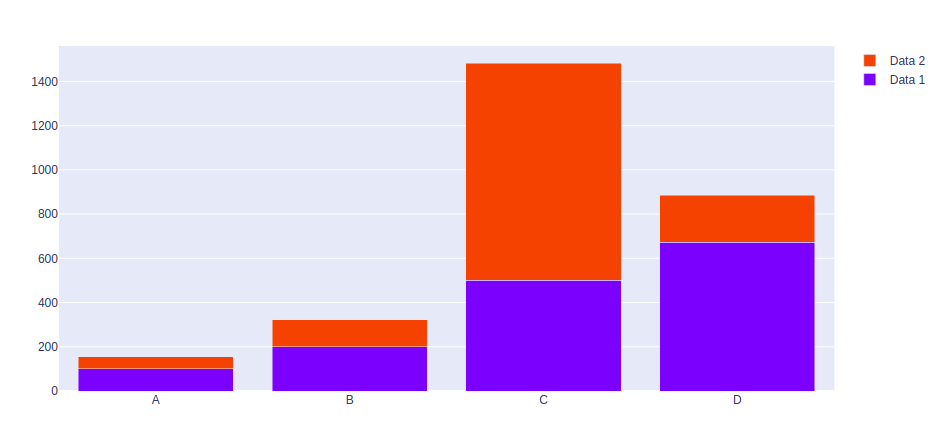# How to create Stacked bar chart in Python-Plotly?

Plotly is a Python library which is used to design graphs, especially interactive graphs. It can plot various graphs and charts like histogram, barplot, boxplot, spreadplot and many more. It is mainly used in data analysis as well as financial analysis. plotly is an interactive visualization library.

## Stack bar chart

A stacked bar chart or  graph is a chart that uses bars to demonstrate comparisons between categories of data, but with ability to impart and compare parts of a whole. Each bar in the chart represents a whole and segments which represent different parts or categories of that whole.

Example 1: Using iris dataset

## Python3

 `import` `plotly.express as px`   `df ``=` `px.data.iris()`   `fig ``=` `px.bar(df, x``=``"sepal_width"``, y``=``"sepal_length"``, color``=``"species"``,` `            ``hover_data``=``[``'petal_width'``], barmode ``=` `'stack'``)`   `fig.show()`

Output:Example 2: Using tips dataset

## Python3

 `import` `plotly.express as px`   `df ``=` `px.data.tips()`   `fig ``=` `px.bar(df, x``=``"total_bill"``, y``=``"day"``, ` `             ``color``=``"sex"``, barmode ``=` `'stack'``)`   `fig.show()`

Output:Example 3: Using graph_objects class

## Python3

 `import` `plotly.graph_objects as px` `import` `numpy as np`     `# creating random data through randomint ` `# function of numpy.random ` `np.random.seed(``42``)`   `random_x``=` `np.random.randint(``1``,``101``,``100``) ` `random_y``=` `np.random.randint(``1``,``101``,``100``)`   `x ``=` `[``'A'``, ``'B'``, ``'C'``, ``'D'``]`   `plot ``=` `px.Figure(data``=``[go.Bar(` `    ``name ``=` `'Data 1'``,` `    ``x ``=` `x,` `    ``y ``=` `[``100``, ``200``, ``500``, ``673``]` `   ``),` `                       ``go.Bar(` `    ``name ``=` `'Data 2'``,` `    ``x ``=` `x,` `    ``y ``=` `[``56``, ``123``, ``982``, ``213``]` `   ``)` `])`   `plot.update_layout(barmode``=``'stack'``)` `                 `  `plot.show()`

Output:Whether you're preparing for your first job interview or aiming to upskill in this ever-evolving tech landscape, GeeksforGeeks Courses are your key to success. We provide top-quality content at affordable prices, all geared towards accelerating your growth in a time-bound manner. Join the millions we've already empowered, and we're here to do the same for you. Don't miss out - check it out now!

Previous
Next